## 3D Transformations in Computer Graphics-

We have discussed-

• Transformation is a process of modifying and re-positioning the existing graphics.
• 3D Transformations take place in a three dimensional plane.

In computer graphics, various transformation techniques are-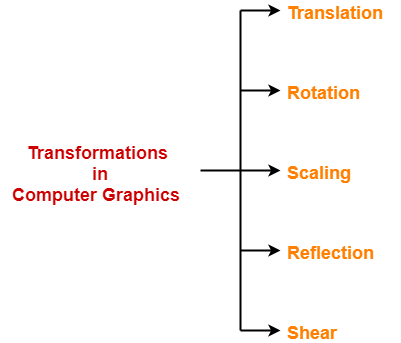## 3D Shearing in Computer Graphics-

 In Computer graphics,3D Shearing is an ideal technique to change the shape of an existing object in a three dimensional plane.

In a three dimensional plane, the object size can be changed along X direction, Y direction as well as Z direction.

So, there are three versions of shearing-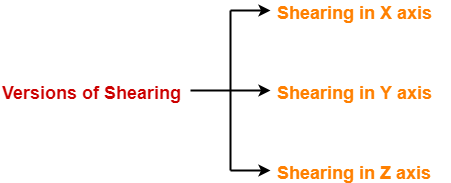1. Shearing in X direction
2. Shearing in Y direction
3. Shearing in Z direction

Consider a point object O has to be sheared in a 3D plane.

Let-

• Initial coordinates of the object O = (Xold, Yold, Zold)
• Shearing parameter towards X direction = Shx
• Shearing parameter towards Y direction = Shy
• Shearing parameter towards Z direction = Shz
• New coordinates of the object O after shearing = (Xnew, Ynew, Znew)

### Shearing in X Axis-

Shearing in X axis is achieved by using the following shearing equations-

• Xnew = Xold
• Ynew = Yold + Shy x Xold
• Znew = Zold + Shz x Xold

In Matrix form, the above shearing equations may be represented as-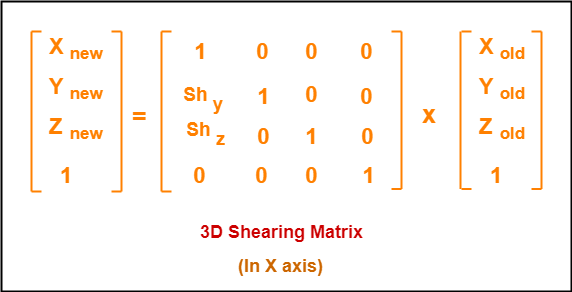### Shearing in Y Axis-

Shearing in Y axis is achieved by using the following shearing equations-

• Xnew = Xold + Shx x Yold
• Ynew = Yold
• Znew = Zold + Shz x Yold

In Matrix form, the above shearing equations may be represented as-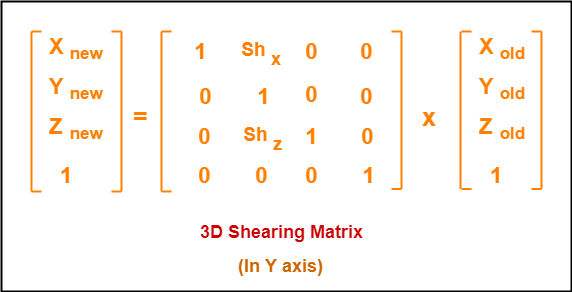### Shearing in Z Axis-

Shearing in Z axis is achieved by using the following shearing equations-

• Xnew = Xold + Shx x Zold
• Ynew = Yold + Shy x Zold
• Znew = Zold

In Matrix form, the above shearing equations may be represented as-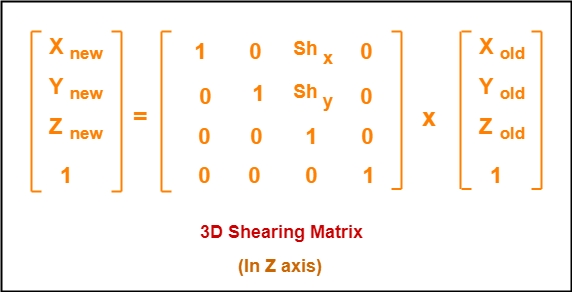## Problem-01:

Given a 3D triangle with points (0, 0, 0), (1, 1, 2) and (1, 1, 3). Apply shear parameter 2 on X axis, 2 on Y axis and 3 on Z axis and find out the new coordinates of the object.

## Solution-

Given-

• Old corner coordinates of the triangle = A (0, 0, 0), B(1, 1, 2), C(1, 1, 3)
• Shearing parameter towards X direction (Shx) = 2
• Shearing parameter towards Y direction (Shy) = 2
• Shearing parameter towards Y direction (Shz) = 3

## Shearing in X Axis-

### For Coordinates A(0, 0, 0)

Let the new coordinates of corner A after shearing = (Xnew, Ynew, Znew).

Applying the shearing equations, we have-

• Xnew = Xold = 0
• Ynew = Yold + Shy x Xold = 0 + 2 x 0 = 0
• Znew = Zold + Shz x Xold = 0 + 3 x 0 = 0

Thus, New coordinates of corner A after shearing = (0, 0, 0).

### For Coordinates B(1, 1, 2)

Let the new coordinates of corner B after shearing = (Xnew, Ynew, Znew).

Applying the shearing equations, we have-

• Xnew = Xold = 1
• Ynew = Yold + Shy x Xold = 1 + 2 x 1 = 3
• Znew = Zold + Shz x Xold = 2 + 3 x 1 = 5

Thus, New coordinates of corner B after shearing = (1, 3, 5).

### For Coordinates C(1, 1, 3)

Let the new coordinates of corner C after shearing = (Xnew, Ynew, Znew).

Applying the shearing equations, we have-

• Xnew = Xold = 1
• Ynew = Yold + Shy x Xold = 1 + 2 x 1 = 3
• Znew = Zold + Shz x Xold = 3 + 3 x 1 = 6

Thus, New coordinates of corner C after shearing = (1, 3, 6).

Thus, New coordinates of the triangle after shearing in X axis = A (0, 0, 0), B(1, 3, 5), C(1, 3, 6).

## Shearing in Y Axis-

### For Coordinates A(0, 0, 0)

Let the new coordinates of corner A after shearing = (Xnew, Ynew, Znew).

Applying the shearing equations, we have-

• Xnew = Xold + Shx x Yold = 0 + 2 x 0 = 0
• Ynew = Yold = 0
• Znew = Zold + Shz x Yold = 0 + 3 x 0 = 0

Thus, New coordinates of corner A after shearing = (0, 0, 0).

### For Coordinates B(1, 1, 2)

Let the new coordinates of corner B after shearing = (Xnew, Ynew, Znew).

Applying the shearing equations, we have-

• Xnew = Xold + Shx x Yold = 1 + 2 x 1 = 3
• Ynew = Yold = 1
• Znew = Zold + Shz x Yold = 2 + 3 x 1 = 5

Thus, New coordinates of corner B after shearing = (3, 1, 5).

### For Coordinates C(1, 1, 3)

Let the new coordinates of corner C after shearing = (Xnew, Ynew, Znew).

Applying the shearing equations, we have-

• Xnew = Xold + Shx x Yold = 1 + 2 x 1 = 3
• Ynew = Yold = 1
• Znew = Zold + Shz x Yold = 3 + 3 x 1 = 6

Thus, New coordinates of corner C after shearing = (3, 1, 6).

Thus, New coordinates of the triangle after shearing in Y axis = A (0, 0, 0), B(3, 1, 5), C(3, 1, 6).

## Shearing in Z Axis-

### For Coordinates A(0, 0, 0)

Let the new coordinates of corner A after shearing = (Xnew, Ynew, Znew).

Applying the shearing equations, we have-

• Xnew = Xold + Shx x Zold = 0 + 2 x 0 = 0
• Ynew = Yold + Shy x Zold = 0 + 2 x 0 = 0
• Znew = Zold = 0

Thus, New coordinates of corner A after shearing = (0, 0, 0).

### For Coordinates B(1, 1, 2)

Let the new coordinates of corner B after shearing = (Xnew, Ynew, Znew).

Applying the shearing equations, we have-

• Xnew = Xold + Shx x Zold = 1 + 2 x 2 = 5
• Ynew = Yold + Shy x Zold = 1 + 2 x 2 = 5
• Znew = Zold = 2

Thus, New coordinates of corner B after shearing = (5, 5, 2).

### For Coordinates C(1, 1, 3)

Let the new coordinates of corner C after shearing = (Xnew, Ynew, Znew).

Applying the shearing equations, we have-

• Xnew = Xold + Shx x Zold = 1 + 2 x 3 = 7
• Ynew = Yold + Shy x Zold = 1 + 2 x 3 = 7
• Znew = Zold = 3

Thus, New coordinates of corner C after shearing = (7, 7, 3).

Thus, New coordinates of the triangle after shearing in Z axis = A (0, 0, 0), B(5, 5, 2), C(7, 7, 3).

To gain better understanding about 3D Shearing in Computer Graphics,

Watch this Video Lecture

Next Article- Bezier Curves

Get more notes and other study material of Computer Graphics.

Watch video lectures by visiting our YouTube channel LearnVidFun.

## 3D Transformations in Computer Graphics-

We have discussed-

• Transformation is a process of modifying and re-positioning the existing graphics.
• 3D Transformations take place in a three dimensional plane.

In computer graphics, various transformation techniques are-## 3D Reflection in Computer Graphics-

• Reflection is a kind of rotation where the angle of rotation is 180 degree.
• The reflected object is always formed on the other side of mirror.
• The size of reflected object is same as the size of original object.

Consider a point object O has to be reflected in a 3D plane.

Let-

• Initial coordinates of the object O = (Xold, Yold, Zold)
• New coordinates of the reflected object O after reflection = (Xnew, Ynew,Znew)

In 3 dimensions, there are 3 possible types of reflection-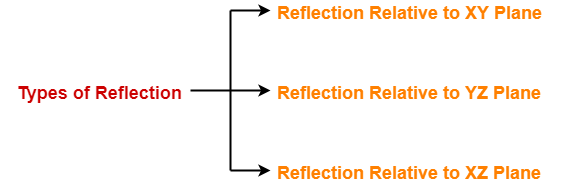• Reflection relative to XY plane
• Reflection relative to YZ plane
• Reflection relative to XZ plane

### Reflection Relative to XY Plane:

This reflection is achieved by using the following reflection equations-

• Xnew = Xold
• Ynew = Yold
• Znew = -Zold

In Matrix form, the above reflection equations may be represented as-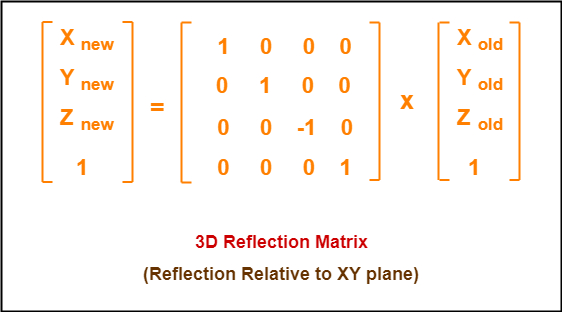### Reflection Relative to YZ Plane:

This reflection is achieved by using the following reflection equations-

• Xnew = -Xold
• Ynew = Yold
• Znew = Zold

In Matrix form, the above reflection equations may be represented as-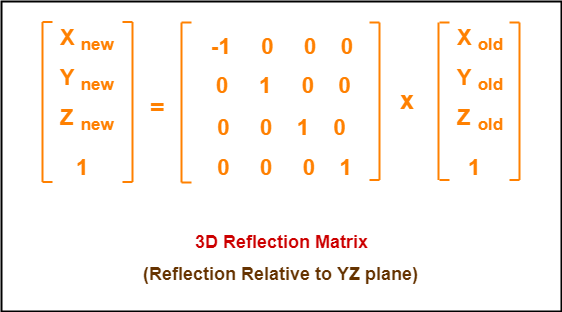### Reflection Relative to XZ Plane:

This reflection is achieved by using the following reflection equations-

• Xnew = Xold
• Ynew = -Yold
• Znew = Zold

In Matrix form, the above reflection equations may be represented as-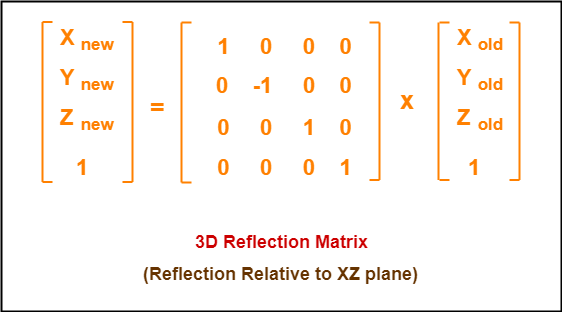## Problem-01:

Given a 3D triangle with coordinate points A(3, 4, 1), B(6, 4, 2), C(5, 6, 3). Apply the reflection on the XY plane and find out the new coordinates of the object.

## Solution-

Given-

• Old corner coordinates of the triangle = A (3, 4, 1), B(6, 4, 2), C(5, 6, 3)
• Reflection has to be taken on the XY plane

### For Coordinates A(3, 4, 1)

Let the new coordinates of corner A after reflection = (Xnew, Ynew, Znew).

Applying the reflection equations, we have-

• Xnew = Xold = 3
• Ynew = Yold = 4
• Znew = -Zold = -1

Thus, New coordinates of corner A after reflection = (3, 4, -1).

### For Coordinates B(6, 4, 2)

Let the new coordinates of corner B after reflection = (Xnew, Ynew, Znew).

Applying the reflection equations, we have-

• Xnew = Xold = 6
• Ynew = Yold = 4
• Znew = -Zold = -2

Thus, New coordinates of corner B after reflection = (6, 4, -2).

### For Coordinates C(5, 6, 3)

Let the new coordinates of corner C after reflection = (Xnew, Ynew, Znew).

Applying the reflection equations, we have-

• Xnew = Xold = 5
• Ynew = Yold = 6
• Znew = -Zold = -3

Thus, New coordinates of corner C after reflection = (5, 6, -3).

Thus, New coordinates of the triangle after reflection = A (3, 4, -1), B(6, 4, -2), C(5, 6, -3).

## Problem-02:

Given a 3D triangle with coordinate points A(3, 4, 1), B(6, 4, 2), C(5, 6, 3). Apply the reflection on the XZ plane and find out the new coordinates of the object.

## Solution-

Given-

• Old corner coordinates of the triangle = A (3, 4, 1), B(6, 4, 2), C(5, 6, 3)
• Reflection has to be taken on the XZ plane

### For Coordinates A(3, 4, 1)

Let the new coordinates of corner A after reflection = (Xnew, Ynew, Znew).

Applying the reflection equations, we have-

• Xnew = Xold = 3
• Ynew = -Yold = -4
• Znew = Zold = 1

Thus, New coordinates of corner A after reflection = (3, -4, 1).

### For Coordinates B(6, 4, 2)

Let the new coordinates of corner B after reflection = (Xnew, Ynew, Znew).

Applying the reflection equations, we have-

• Xnew = Xold = 6
• Ynew = -Yold = -4
• Znew = Zold = 2

Thus, New coordinates of corner B after reflection = (6, -4, 2).

### For Coordinates C(5, 6, 3)

Let the new coordinates of corner C after reflection = (Xnew, Ynew, Znew).

Applying the reflection equations, we have-

• Xnew = Xold = 5
• Ynew = -Yold = -6
• Znew = Zold = 3

Thus, New coordinates of corner C after reflection = (5, -6, 3).

Thus, New coordinates of the triangle after reflection = A (3, -4, 1), B(6, -4, 2), C(5, -6, 3).

To gain better understanding about 3D Reflection in Computer Graphics,

Watch this Video Lecture

Next Article- 3D Shearing in Computer Graphics

Get more notes and other study material of Computer Graphics.

Watch video lectures by visiting our YouTube channel LearnVidFun.

## 3D Transformations in Computer Graphics-

We have discussed-

• Transformation is a process of modifying and re-positioning the existing graphics.
• 3D Transformations take place in a three dimensional plane.

In computer graphics, various transformation techniques are-## 3D Scaling in Computer Graphics-

 In computer graphics, scaling is a process of modifying or altering the size of objects.

• Scaling may be used to increase or reduce the size of object.
• Scaling subjects the coordinate points of the original object to change.
• Scaling factor determines whether the object size is to be increased or reduced.
• If scaling factor > 1, then the object size is increased.
• If scaling factor < 1, then the object size is reduced.

Consider a point object O has to be scaled in a 3D plane.

Let-

• Initial coordinates of the object O = (Xold, Yold,Zold)
• Scaling factor for X-axis = Sx
• Scaling factor for Y-axis = Sy
• Scaling factor for Z-axis = Sz
• New coordinates of the object O after scaling = (Xnew, Ynew, Znew)

This scaling is achieved by using the following scaling equations-

• Xnew = Xold x Sx
• Ynew = Yold x Sy
• Znew = Zold x Sz

In Matrix form, the above scaling equations may be represented as-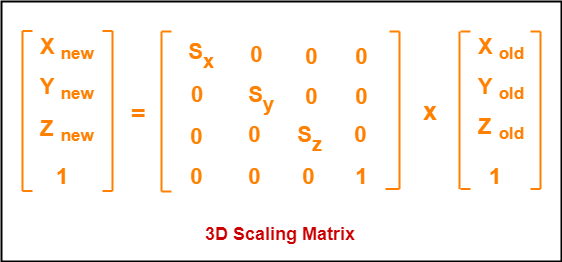## Problem-01:

Given a 3D object with coordinate points A(0, 3, 3), B(3, 3, 6), C(3, 0, 1), D(0, 0, 0). Apply the scaling parameter 2 towards X axis, 3 towards Y axis and 3 towards Z axis and obtain the new coordinates of the object.

## Solution-

Given-

• Old coordinates of the object  = A (0, 3, 3), B(3, 3, 6), C(3, 0, 1), D(0, 0, 0)
• Scaling factor along X axis = 2
• Scaling factor along Y axis = 3
• Scaling factor along Z axis = 3

### For Coordinates A(0, 3, 3)

Let the new coordinates of A after scaling = (Xnew, Ynew, Znew).

Applying the scaling equations, we have-

• Xnew = Xold x Sx = 0  x 2 = 0
• Ynew = Yold x Sy = 3 x 3 = 9
• Znew = Zold x Sz = 3 x 3 = 9

Thus, New coordinates of corner A after scaling = (0, 9, 9).

### For Coordinates B(3, 3, 6)

Let the new coordinates of B after scaling = (Xnew, Ynew, Znew).

Applying the scaling equations, we have-

• Xnew = Xold x Sx = 3  x 2 = 6
• Ynew = Yold x Sy = 3 x 3 = 9
• Znew = Zold x Sz = 6 x 3 = 18

Thus, New coordinates of corner B after scaling = (6, 9, 18).

### For Coordinates C(3, 0, 1)

Let the new coordinates of C after scaling = (Xnew, Ynew, Znew).

Applying the scaling equations, we have-

• Xnew = Xold x Sx = 3  x 2 = 6
• Ynew = Yold x Sy = 0 x 3 = 0
• Znew = Zold x Sz = 1 x 3 = 3

Thus, New coordinates of corner C after scaling = (6, 0, 3).

### For Coordinates D(0, 0, 0)

Let the new coordinates of D after scaling = (Xnew, Ynew, Znew).

Applying the scaling equations, we have-

• Xnew = Xold x Sx = 0  x 2 = 0
• Ynew = Yold x Sy = 0 x 3 = 0
• Znew = Zold x Sz = 0 x 3 = 0

Thus, New coordinates of corner D after scaling = (0, 0, 0).

To gain better understanding about 3D Scaling in Computer Graphics,

Watch this Video Lecture

Next Article- 3D Reflection in Computer Graphics

Get more notes and other study material of Computer Graphics.

Watch video lectures by visiting our YouTube channel LearnVidFun.

## 3D Transformations in Computer Graphics-

We have discussed-

• Transformation is a process of modifying and re-positioning the existing graphics.
• 3D Transformations take place in a three dimensional plane.

In computer graphics, various transformation techniques are-## 3D Rotation in Computer Graphics-

 In Computer graphics,3D Rotation is a process of rotating an object with respect to an angle in a three dimensional plane.

Consider a point object O has to be rotated from one angle to another in a 3D plane.

Let-

• Initial coordinates of the object O = (Xold, Yold, Zold)
• Initial angle of the object O with respect to origin = Φ
• Rotation angle = θ
• New coordinates of the object O after rotation = (Xnew, Ynew, Znew)

In 3 dimensions, there are 3 possible types of rotation-

• X-axis Rotation
• Y-axis Rotation
• Z-axis Rotation

### For X-Axis Rotation-

This rotation is achieved by using the following rotation equations-

• Xnew = Xold
• Ynew = Yold x cosθ – Zold x sinθ
• Znew = Yold x sinθ + Zold x cosθ

In Matrix form, the above rotation equations may be represented as-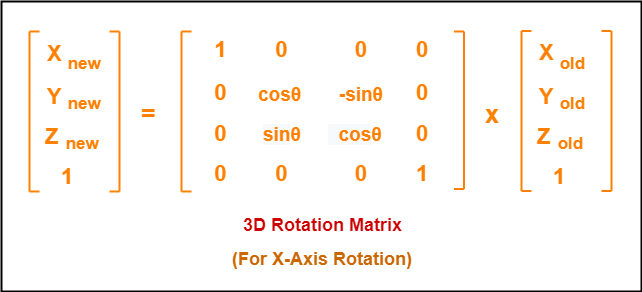### For Y-Axis Rotation-

This rotation is achieved by using the following rotation equations-

• Xnew = Zold x sinθ + Xold x cosθ
• Ynew = Yold
• Znew = Yold x cosθ – Xold x sinθ

In Matrix form, the above rotation equations may be represented as-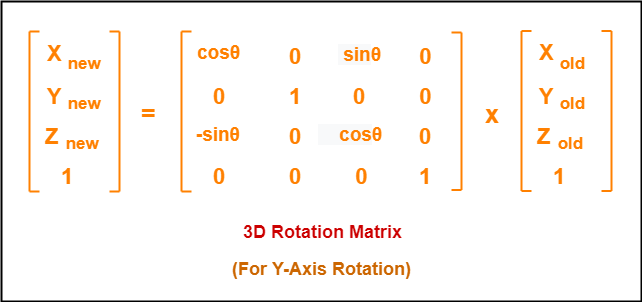### For Z-Axis Rotation-

This rotation is achieved by using the following rotation equations-

• Xnew = Xold x cosθ – Yold x sinθ
• Ynew = Xold x sinθ + Yold x cosθ
• Znew = Zold

In Matrix form, the above rotation equations may be represented as-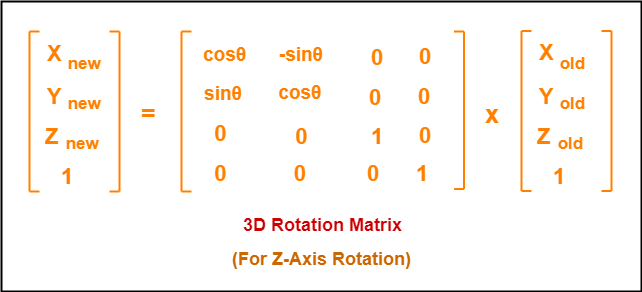## Problem-01:

Given a homogeneous point (1, 2, 3). Apply rotation 90 degree towards X, Y and Z axis and find out the new coordinate points.

## Solution-

Given-

• Old coordinates = (Xold, Yold, Zold) = (1, 2, 3)
• Rotation angle = θ = 90º

### For X-Axis Rotation-

Let the new coordinates after rotation = (Xnew, Ynew, Znew).

Applying the rotation equations, we have-

• Xnew = Xold = 1
• Ynew = Yold x cosθ – Zold x sinθ = 2 x cos90° – 3 x sin90° = 2 x 0 – 3 x 1 = -3
• Znew = Yold x sinθ + Zold x cosθ = 2 x sin90° + 3 x cos90° = 2 x 1 + 3 x 0 = 2

Thus, New coordinates after rotation = (1, -3, 2).

### For Y-Axis Rotation-

Let the new coordinates after rotation = (Xnew, Ynew, Znew).

Applying the rotation equations, we have-

• Xnew = Zold x sinθ + Xold x cosθ = 3 x sin90° + 1 x cos90° = 3 x 1 + 1 x 0 = 3
• Ynew = Yold = 2
• Znew = Yold x cosθ – Xold x sinθ = 2 x cos90° – 1 x sin90° = 2 x 0 – 1 x 1 = -1

Thus, New coordinates after rotation = (3, 2, -1).

### For Z-Axis Rotation-

Let the new coordinates after rotation = (Xnew, Ynew, Znew).

Applying the rotation equations, we have-

• Xnew = Xold x cosθ – Yold x sinθ = 1 x cos90° – 2 x sin90° = 1 x 0 – 2 x 1 = -2
• Ynew = Xold x sinθ + Yold x cosθ = 1 x sin90° + 2 x cos90° = 1 x 1 + 2 x 0 = 1
• Znew = Zold = 3

Thus, New coordinates after rotation = (-2, 1, 3).

To gain better understanding about 3D Rotation in Computer Graphics,

Watch this Video Lecture

Next Article- 3D Scaling in Computer Graphics

Get more notes and other study material of Computer Graphics.

Watch video lectures by visiting our YouTube channel LearnVidFun.

## 3D Transformation in Computer Graphics-

 In Computer graphics,Transformation is a process of modifying and re-positioning the existing graphics.

• 3D Transformations take place in a three dimensional plane.
• 3D Transformations are important and a bit more complex than 2D Transformations.
• Transformations are helpful in changing the position, size, orientation, shape etc of the object.

### Transformation Techniques-

In computer graphics, various transformation techniques are-## 3D Translation in Computer Graphics-

 In Computer graphics,3D Translation is a process of moving an object from one position to another in a three dimensional plane.

Consider a point object O has to be moved from one position to another in a 3D plane.

Let-

• Initial coordinates of the object O = (Xold, Yold, Zold)
• New coordinates of the object O after translation = (Xnew, Ynew, Zold)
• Translation vector or Shift vector = (Tx, Ty, Tz)

Given a Translation vector (Tx, Ty, Tz)-

• Tx defines the distance the Xold coordinate has to be moved.
• Ty defines the distance the Yold coordinate has to be moved.
• Tz defines the distance the Zold coordinate has to be moved.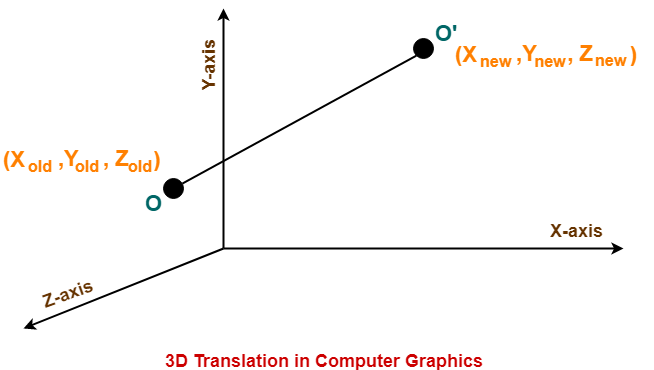This translation is achieved by adding the translation coordinates to the old coordinates of the object as-

• Xnew = Xold + Tx     (This denotes translation towards X axis)
• Ynew = Yold + Ty     (This denotes translation towards Y axis)
• Znew = Zold + Tz     (This denotes translation towards Z axis)

In Matrix form, the above translation equations may be represented as-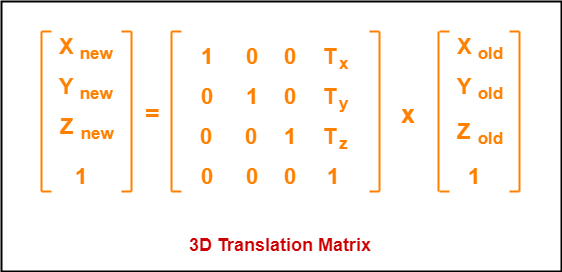Also Read- 2D Translation in Computer Graphics

## Problem-

Given a 3D object with coordinate points A(0, 3, 1), B(3, 3, 2), C(3, 0, 0), D(0, 0, 0). Apply the translation with the distance 1 towards X axis, 1 towards Y axis and 2 towards Z axis and obtain the new coordinates of the object.

## Solution-

Given-

• Old coordinates of the object = A (0, 3, 1), B(3, 3, 2), C(3, 0, 0), D(0, 0, 0)
• Translation vector = (Tx, Ty, Tz) = (1, 1, 2)

### For Coordinates A(0, 3, 1)

Let the new coordinates of A = (Xnew, Ynew, Znew).

Applying the translation equations, we have-

• Xnew = Xold + Tx = 0 + 1 = 1
• Ynew = Yold + Ty = 3 + 1 = 4
• Znew = Zold + Tz = 1 + 2 = 3

Thus, New coordinates of A = (1, 4, 3).

### For Coordinates B(3, 3, 2)

Let the new coordinates of B = (Xnew, Ynew, Znew).

Applying the translation equations, we have-

• Xnew = Xold + Tx = 3 + 1 = 4
• Ynew = Yold + Ty = 3 + 1 = 4
• Znew = Zold + Tz = 2 + 2 = 4

Thus, New coordinates of B = (4, 4, 4).

### For Coordinates C(3, 0, 0)

Let the new coordinates of C = (Xnew, Ynew, Znew).

Applying the translation equations, we have-

• Xnew = Xold + Tx = 3 + 1 = 4
• Ynew = Yold + Ty = 0 + 1 = 1
• Znew = Zold + Tz = 0 + 2 = 2

Thus, New coordinates of C = (4, 1, 2).

### For Coordinates D(0, 0, 0)

Let the new coordinates of D = (Xnew, Ynew, Znew).

Applying the translation equations, we have-

• Xnew = Xold + Tx = 0 + 1 = 1
• Ynew = Yold + Ty = 0 + 1 = 1
• Znew = Zold + Tz = 0 + 2 = 2

Thus, New coordinates of D = (1, 1, 2).

Thus, New coordinates of the object = A (1, 4, 3), B(4, 4, 4), C(4, 1, 2), D(1, 1, 2).

To gain better understanding about 3D Translation in Computer Graphics,

Watch this Video Lecture

Next Article- 3D Rotation in Computer Graphics

Get more notes and other study material of Computer Graphics.

Watch video lectures by visiting our YouTube channel LearnVidFun.